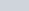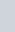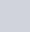Multiplication rule

In this video I prove to you the following result for the multiplication of two complex numbers when given in modulus-argument form:

Rule to remember:

•Multiplication Rule for the Mod-Arg of two Complex Numbers : ExamSolutions Maths Tutorials - youtube Video

Division rule

In this video I prove to you the following result for the division of two complex numbers when given in modulus-argument form :

Rule to remember:

•Division Rule for the Mod-Arg of two Complex Numbers : ExamSolutions Maths Tutorials - youtube Video

Mixed Examples

Example:1.2.3.Multiplication & Division of Complex Numbers in Mod-Arg form Examples : ExamSolutions - youtube Video